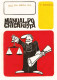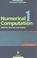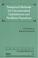### Computer Aided Analysis and Design

#### Course Description

The role of computers in analysis and design of technical systems is presented. The course describes error handling in computer calculations, linear system solving algorithms and numerical optimization algorithms, as well as evolutionary algorithms. The transient analysis formulations and methods are presented. The systems behaviour is analysed in regard of system stability and chaotic properties. The presented algorithms are evaluated in regard of their computational efficiency.

#### General Competencies

Students will be able to determine the floating number precision impact on the correctness of computation algorithms. Students would also apply different deterministic and stochastic optimization methods to the problem at hand. Individual projects include implementation and usage of linear systems decomposition methods and various optimization algorithms. Additionally, students will be introduced to the usage of an engineering simulation and calculation tool such as MATLAB.

#### Learning Outcomes

1. recognize an optimization problem
2. apply a nonlinear optimization procedure
3. compare different optimization algorithms
4. define floating point precision standard
5. describe the problem of solving differential equations systems
6. apply a numerical integration method
7. compare the precision and stability of numerical integration methods

#### Forms of Teaching

Lectures

Teaching is organized into two teaching cycles (15-week classes). First cycle consists of 7-week classes and midterm exam. Second cycle consists of 6-week classes and final exam.

Exams

Midterm, final exam, homework

Exercises

Additional examples are provided.

Consultations

Consultation hours will be given at the first lecture

Programming Exercises

homework

#### Grading Method

Continuous Assessment Exam
Type Threshold Percent of Grade Threshold Percent of Grade
Homeworks 0 % 25 % 0 % 25 %
Mid Term Exam: Written 0 % 30 % 0 %
Final Exam: Written 0 % 45 %
Exam: Written 50 % 40 %
Exam: Oral 35 %
##### Comment:

All homework assignments must be handed in (regardless of the number of points) as a condition for taking the exam

#### Week by Week Schedule

1. Introduction. Algorithms for matrix manipulation. Solving linear equation systems.
2. Permutation matrices. Decomposition algorithms.
3. Matrix inversion. Engineering tools.
4. Optimization problems and algorithms. Definition of goal function.
5. Algorithms for unimodal interval reduction.
6. Multiple variable nonlinear function optimization methods. Unconstrained optimization.
7. Constrained optimization.
8. (Midterm)
9. Stochastic optimization algorithms. Genetic algorithm.
10. Algorithm complexity. Definition of complexity measures.
11. Numerical transient analysis.
12. Stalibity and accurateness of solving algorithms.
13. Explicit and implicit methods. Linear multistep methods.
14. Explicit and implicit methods. Linear multistep methods.
15. (Final exam)

#### Study Programmes

##### University graduate
Computer Engineering (profile)
Specialization Course (1. semester) (3. semester)
Computer Science (profile)
Specialization Course (3. semester) Theoretical Course (1. semester)
Software Engineering and Information Systems (profile)
Specialization Course (1. semester) (3. semester)
Telecommunication and Informatics (profile)
Recommended elective courses (3. semester)

#### Literature

S. Turk, L. Budin (1989.), Analiza i projektiranje računalom, Školska knjiga, ZagrebL. Budin (2002.), Analiza i projektiranje računalom - skripta, FERC. W. Ueberhuber (1995.), Numerical Computation, Springer, ViennaJ. E. Dennis, R. B. Schnabel (1996.), Numerical Methods for Unconstrained Optimization and Nonlinear Equations, SIAM, Philadelphia

#### General

ID 85975
Winter semester
5 ECTS
L0 English Level
L1 e-Learning
45 Lectures
0 Exercises
0 Laboratory exercises
0 Project laboratory

88 Excellent
75 Very Good
63 Good
50 Acceptable